# Bar Graphs

Bar Graphs

Peter wanted to arrange the birthdays of his classmates according to their months.

He then wants to display the data in a way that he can visually understand them.

This is where he faces a problem.

He doesn't want to use a pictograph since it takes too much time also might be difficult.

He wants to do it in an easier method.

Can you suggest a better option for Peter?

Bar graphs can help him represent the data using rectangular bars, aiding better visualization.

In this chapter, we will learn about the types of bar graphs and double bar graphs. Check out the interactive simulation to know more about the concept of Bar Graph/ Bar Chart and try your hand at solving a few interesting practice questions at the end of the page.

## Lesson Plan

 1 What is Bar Graph? 2 Important Notes on Bar Graph 3 Solved Examples on Bar Graph 4 Tips and Tricks 5 Interactive Questions on Bar Graph

## What is Bar Graph?

Bar graph is a specific way of representing data using rectangular bars where the length of each bar is proportional to the value they represent.

Bar graphs are the pictorial representation of grouped data and are also referred to as bar charts.

Bar graphs are one of the examples of data handling.

Bar graphs are an excellent tool when representing data that are independent of one another and don’t need to be in any specific order while being represented.

The bars provide a visual display for comparing quantities in different categories.

The graphs can be plotted vertically (bars standing up) or horizontally (bars laying flat from left to right), but normally we use vertical bars.

Example:

Liza went to the market for buying different types of fruits. She then wants to display the data in a way that she can visually understand which type of fruits she buys the most.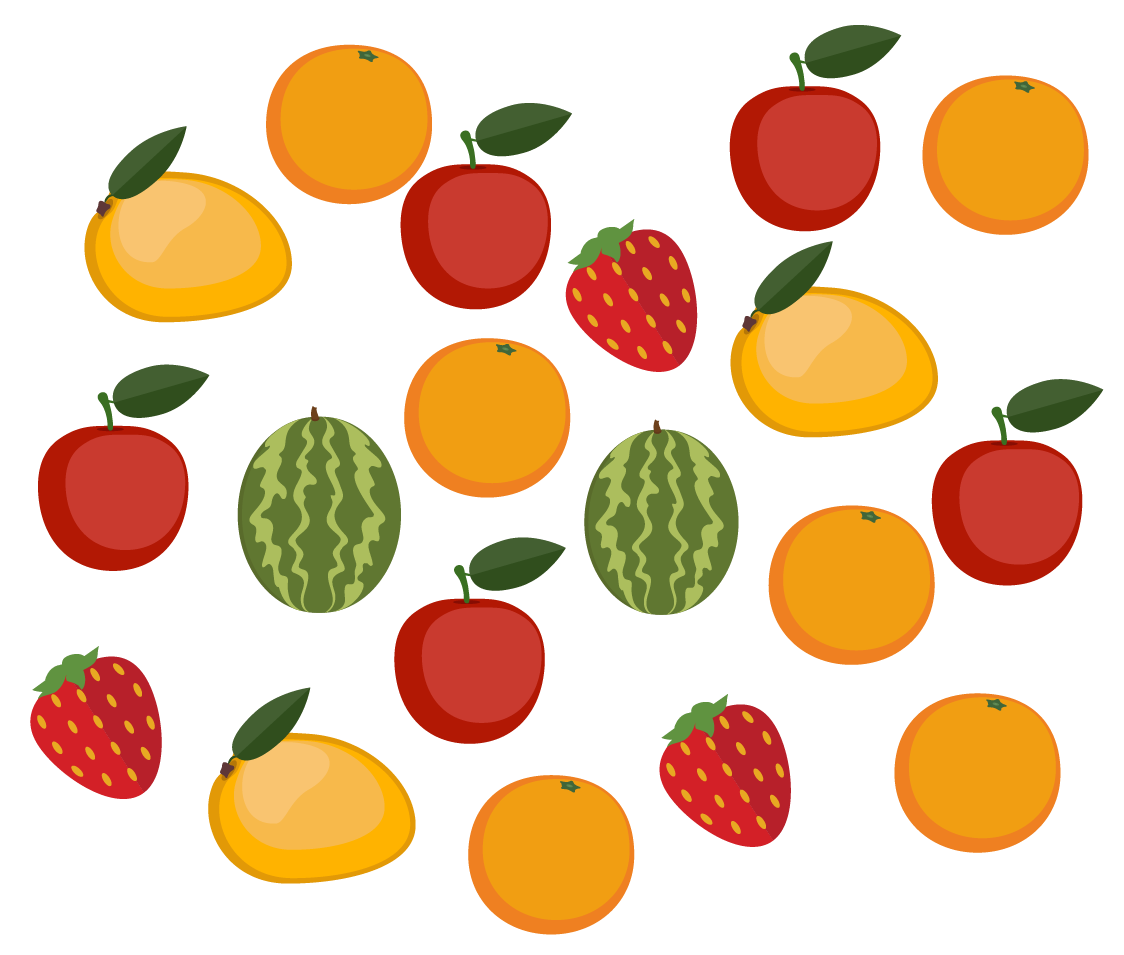We can start by counting each type of fruit and putting the data in a table.

Types of Fruits

Number of Fruits

Apples

5

Mangoes

3

Watermelons

2

Strawberries

3

Oranges

6

Let’s represent the data given below using a bar graph.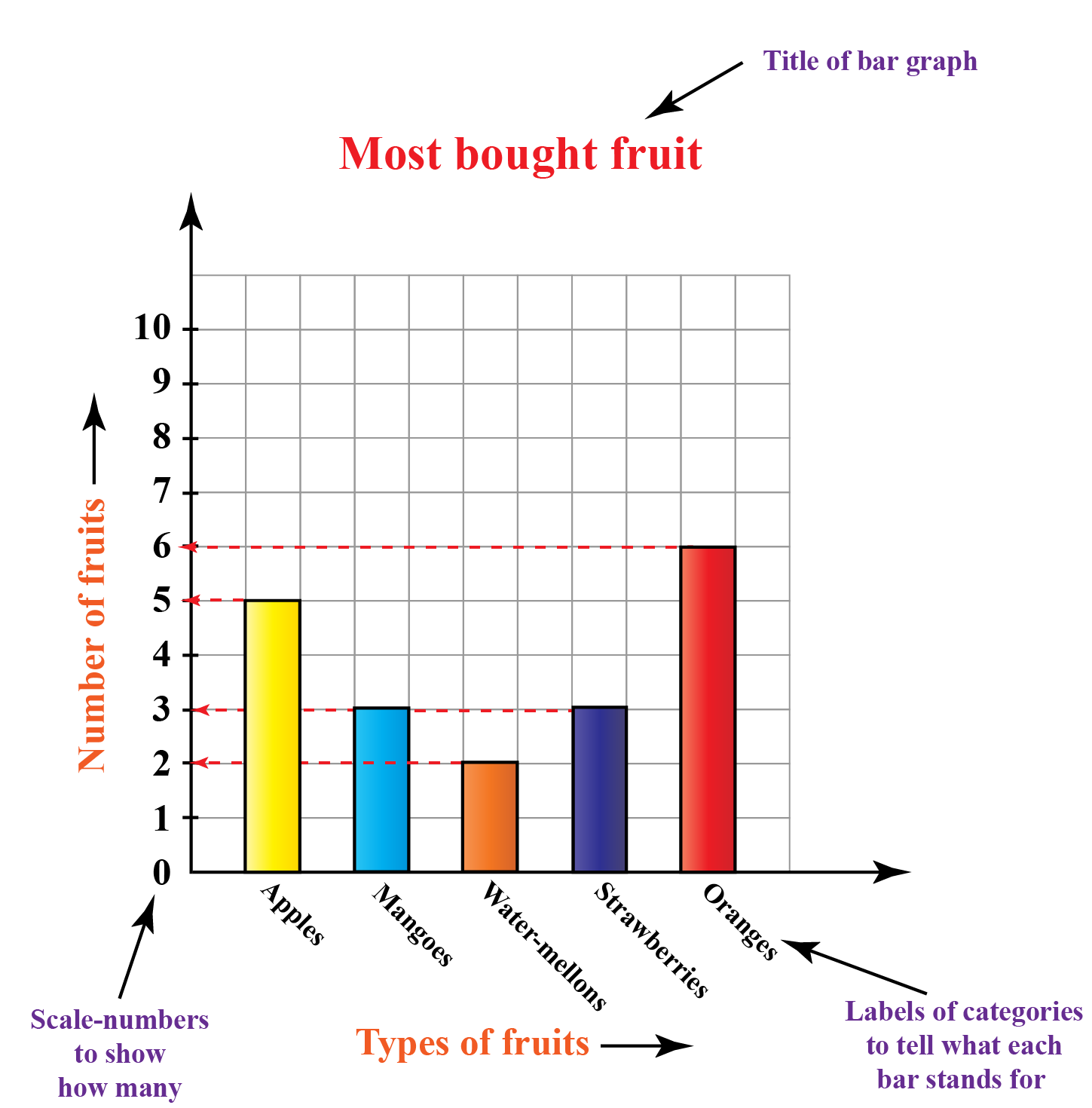Now, the above bar graph shows the different types of fruits and the number of fruits bought by Liza.

From the above graph, we can easily say that orange is the type of fruit that Liza buys the most by looking.

This is the advantage of bar graphs.

### Properties of Bar Graph

• All bars must be of equal width.
• The bars can be drawn horizontally as well as vertically as per your needs.
• The height of the bar is proportional to the data they represent.
• All bars must share a common base.

## Types of Bar Graphs

To presenting the data visually, there are several different types of bar graphs we can consider.

There are different types of bar graphs and they are as follows:

1. Vertical bar graph
2. Horizontal bar graph

### 1. Vertical Graph

The most common type of bar graph is the vertical bar graph. It is very useful when representing a series of data over time.

"The bar graphs that represent the grouped data vertically are called vertical bar graphs."

The horizontal (x)-axis represents the categories and the vertical (y)-axis represents the data value for those categories.

This is how a vertical graph looks like: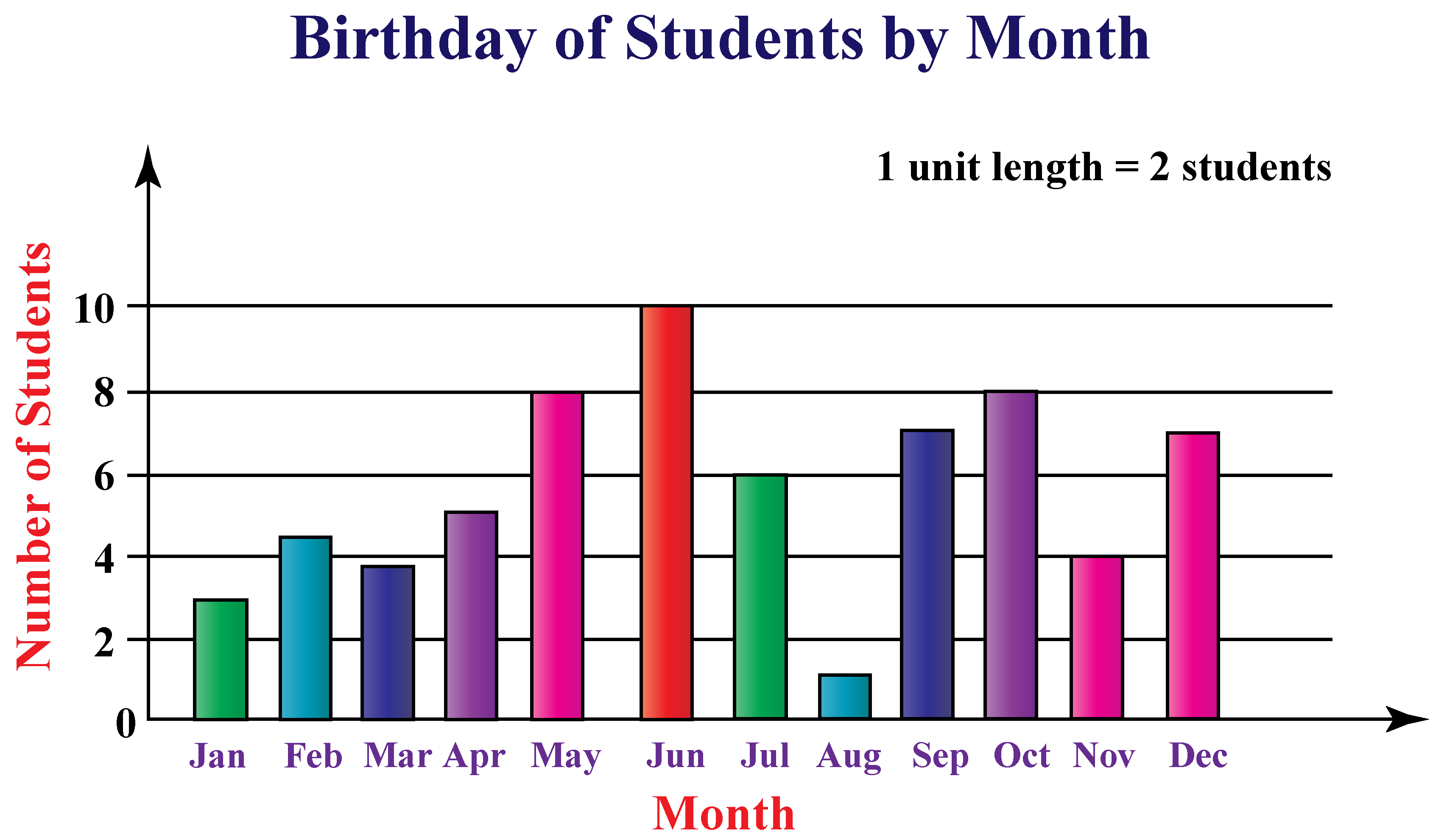### 2. Horizontal Graph

"The bar graphs that represent the grouped data horizontally are called horizontal bar graphs."

The data categories are shown on the vertical axis and the data values are shown on the horizontal axis.

This is how a horizontal graph looks like: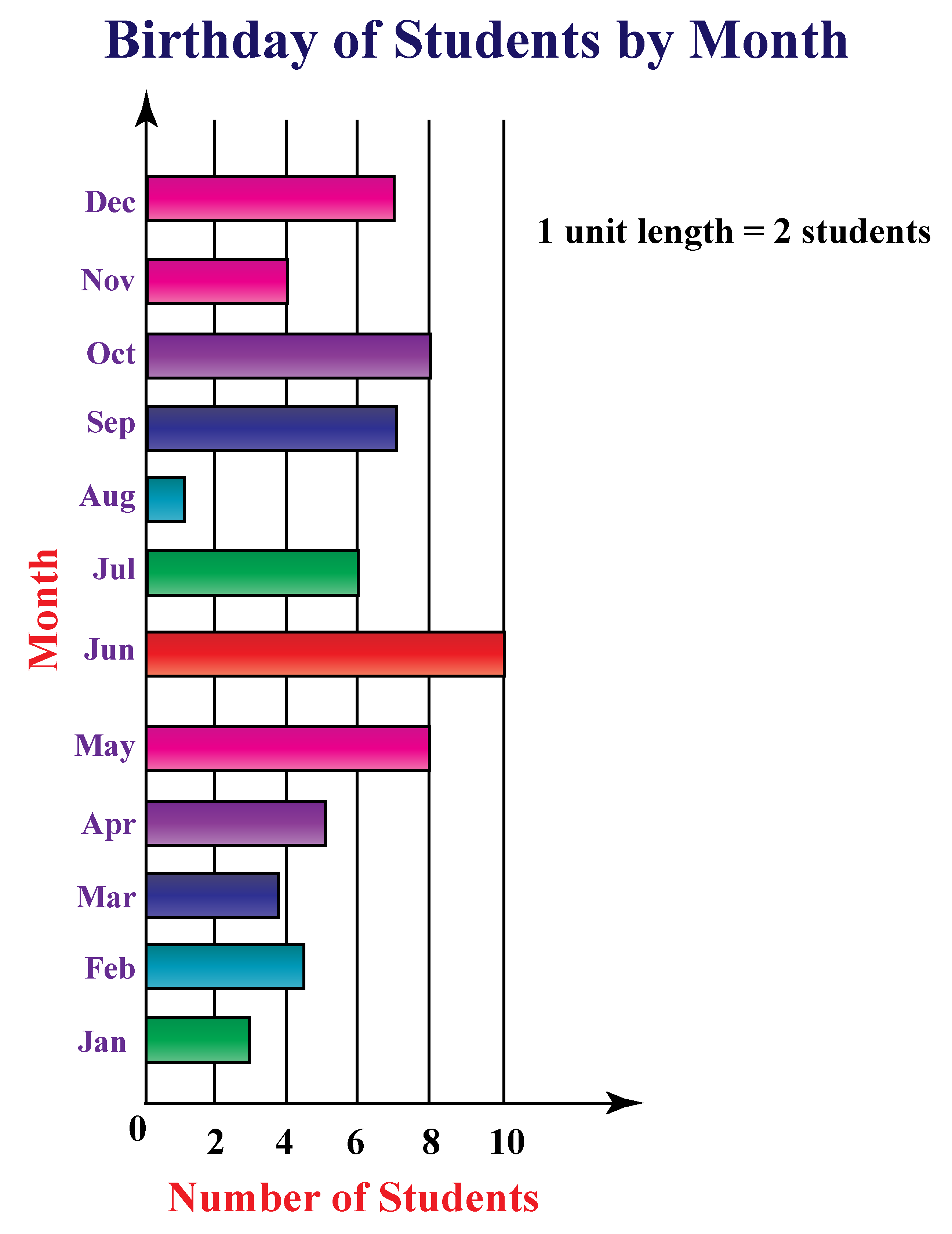### Double bar graph

A double bar graph is just like a normal bar graph. The only difference is that it is used to compares 2 data groups.

In other words, a double bar graph is a graphical representation of information using two bars beside each other at various heights.

The bars can be arranged vertically or horizontally.

It is also referred to as double bar charts.

For example:

The sales of English and Science books in the years  2008, 2009, 2010, 2011 and 2012 are given below in the table:

Years

2015

2016

2017

2018

2019

English

30

35

20

25

40

Science

20

40

25

35

20

Let’s represent the data given above using a double bar graph.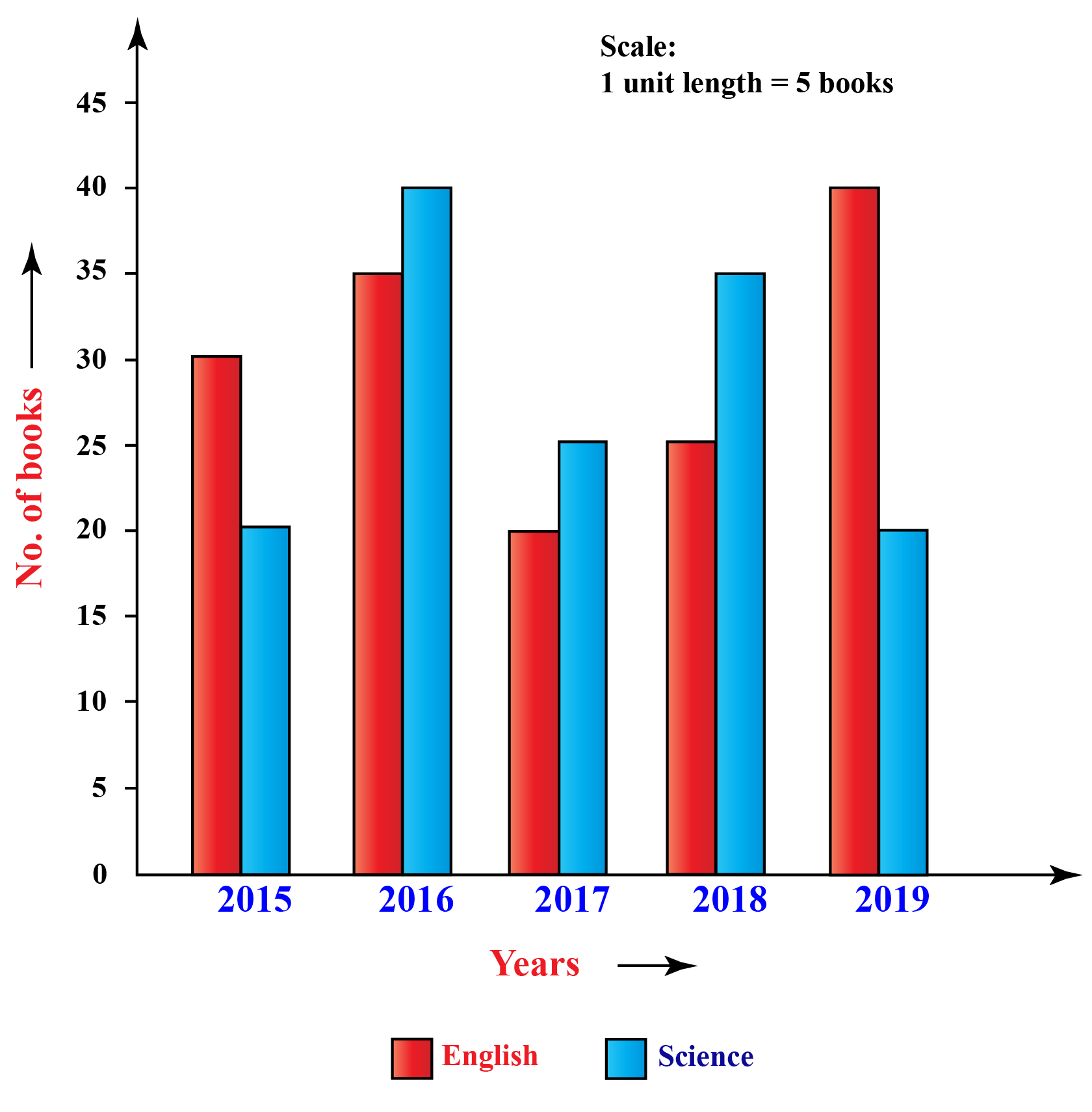More Important Topics
Numbers
Algebra
Geometry
Measurement
Money
Data
Trigonometry
Calculus